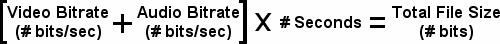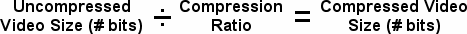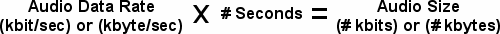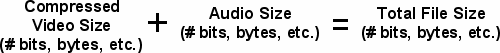#### How much space do I need for my Video?

Digital video usually requires large amounts of hard drive space. However, it is sometimes difficult to determine just how much space you’ll need. Depending on the video format and the encoding used, the amount of space required can vary greatly. The following will explain how to calculate data rates and file sizes.

### What is a Data Rate?

A data rate is the rate at which information being transferred. It’s expressed in terms of amount of information transferred per unit of time. So when describing the speed of a hard drive we talk in terms of Megabytes/second. Whereas compressed audio files are generally expressed in kilobytes/second. When you know the data rate at which your information is being transferred, it’s possible to determine how much storage you will require on your hard drive.

Bitrate-Based Formats

Popular formats store data as a bitrate, for example MPEG-4 (MP4) and Windows Media Video (WMV).

To calculate the file size of a bitrate you’ll need to know three things: the video bitrate, the audio bitrate (if your video has a soundtrack), and the length of the video.

The formula is as follows:The number of bits can be shown as either single bits, kilobits, or Megabits. Once you have the total file size (in bits, kilobits, etc.), you’ll probably want to convert it to Megabytes or Gigabytes. To do this you need to convert from bits to bytes and from the original prefix (kilo, etc.) to Mega or Giga.

For example, here’s how you would convert 36,500,000 bits to Megabytes:

1) 36,500,000 [bits] divided by (8) [bits/byte] = 4,562,500 bytes
2) 4,562,500 bytes divided by (1,048,576) [bytes/Megabyte] = ~ 4.35 Megabytes

Quality/Ratio-Based Formats

Quality-based formats (or ratio-based) are common in digital video capture and editing. Instead of compressing a video to a specified bitrate, they compress the video to a certain ratio of its original size. The compression ratio depends on a variety of factors, such as the video encoding, the quality of the video, and the format of the video. However, for a given video and encoding codec, the ratio is relatively constant but still not necessarily easy to calculate without running the file!

### To calculate the file size of a Quality/Ratio-Based format:

This is a four-step process.
First, you must determine the size the video would have if it had no compression.
Second, you have to apply a compression ratio to the uncompressed video size to obtain the compressed video size.
Third, you must calculate the size of the audio data.
Forth, you simply add the audio and video sizes together.

*The formulas to do this are below. *

To figure out the uncompressed video size:The number of bits/pixel will depend on your video’s colour format. For example, YUY2 and UYVY have 16 bits/pixel and RGB24 has 24 bits/pixel.

Now apply the video compression ratio:The compression ratio will depend on the video codec, the quality of the video, and the format of the video.
You can determine the compression ratio by creating a short clip and calculating its compression ratio.
The compression ratio = (uncompressed video size divided by the compressed video size).

Now determine the size of the audio data using this formula:Windows usually shows you the audio data rate in kilobytes/second when you choose your audio format.
For example, uncompressed stereo 16-bit 44.1 KHz PCM audio has a data rate of 172 kilobytes/second.
Some audio formats, like MP3, refer to the data rate in terms of kilobits/second. Make sure you don’t mix up bits and bytes.

Finally, you have to make sure that your video and audio sizes are in the same units (bits, kilobytes, etc.).

Then you can just add them up:Once you have the total file size (in bits, or bytes), you can convert it to Megabytes, or Gigabytes.

Compression Ratio Tables:

## Conversion Table: 8 bits = 1 byte 1 kilo(bit/byte) = 1,024 (bits/bytes) 1 Mega(bit/byte) = 1,048,576 (bits/bytes) 1 Giga(bit/byte) = 1,073,741,824 (bits/bytes)

Happy calculating!